Using JMP > Enter and Edit Your Data > Restructure Data > Transform Columns in a JMP Platform
Publication date: 11/10/2021

## Transform Columns in a JMP Platform

Each launch window in JMP enables you to create one or more temporary transform columns for use in performing analyses. These transform columns are not part of the source data table and only can be used within the context of the current launch window. Transform columns use formulas or calculations to define the column values. Closing the launch window deletes any transform columns.

Each column listed in the Select Columns pane of the launch window includes an icon representing the column’s modeling type (continuous, ordinal, or nominal) and the column name. Right-click a column name to create a transform column using Transform, Character, Combine, Pairwise, Aggregate, Distributional, Date Time, Random, Row, Vector, or Formula to calculate the column’s values.

Right-click options depend on the selected column’s data type and number of columns selected.

Figure 4.42 Example of Transform Column Menu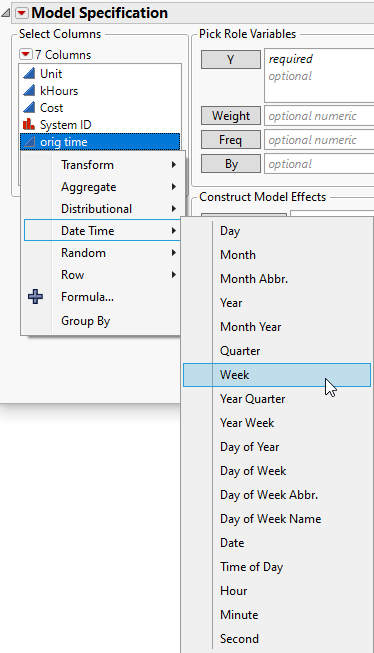Group By

For ordinal and nominal data, specifies the column to use for grouping data. A separate analysis is computed for each level of the specified column.

Notes:

The transform column is available only in the current launch window. To make the transform column available outside of the current launch window, right-click the transform column and select Add to Data Table. The transform column is added to the source data table.

You can paste a transform column into a Roles box on the launch window. For example, you might copy a transform column from a script. Right-click in the appropriate launch window Cast Selected Columns into Roles box and select Paste. This is an alternative to right-clicking the column in the Select Columns list, selecting the transform, and adding the transform column to a role.

If the transform function is invertible, the following platforms use the original scale for the prediction profiler and to save the predicted values and formulas.

Fit Y by X

Standard Least Squares

Partition

Boosted Tree

Bootstrap Forest

Define your own transform by writing a JSL script. See Create Custom Functions, Transforms, and Formats in the Scripting Guide.

Select a function from the Transform menu to create a transform column containing the calculations based on the selected function. See the Scripting Index in the Help menu or Transform in Fitting Linear Models.

Note: You can apply unary functions to multiple columns resulting in multiple transform columns.

 Round Rounds date values. For example, in a chart of weeks, Round converts the date values into the first date of each week that they occur on. Scale Offset Enables you to specify a general linear transform. For example, you might multiply the value by 1.8 and apply an offset of 32 to convert Celsius temperatures to Fahrenheit. Custom Binning Opens the binning window which enables you to distribute the data into bins. Square Root Takes the square root of the values of the selected column. Square Calculates the square for the selected column values. Log Applies the natural logarithm transformation to the selected column. Log x+1 Calculates Log(col+1). Exp Applies the exponential transformation to the selected column. Log10 Applies the base-10 logarithm transformation to the selected column. Pow10 Calculates 10 raised to the power of the selected column values. Cube Root Calculates the cube root for the selected column values. Cube Calculates the cube for the selected column values. Reciprocal Calculates the reciprocal (1/column) for the selected column values. Absolute Value Calculates the absolute value for the selected column values. Negation Calculates the negative for the selected column values. Arrhenius Applies the Arrhenius transformation to the variable T (temperature in degrees Centigrade):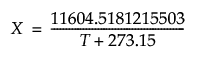This is the component of the Arrhenius relationship that is multiplied by the activation energy. Arrhenius Inverse Applies the inverse of the Arrhenius transformation to the variable X: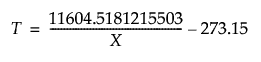Logit Calculates the inverse of the logistic function for the selected column (where p is in the range of 0 to 1):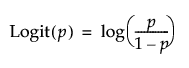Logistic Calculates the logistic (also known as Squish and Logist) function for the selected column (where the result is in the range of 0 to 1):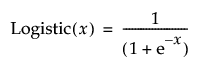Logit Percent Calculates the logit as a percent for the selected column (where pct is a percent in the range of 0 to 100):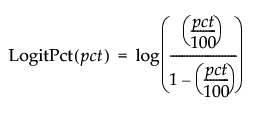Logistic Percent Calculates the logistic (or logist) as a percent for the selected column (where the result is in the range of 0 to 100):Select multiple columns to access the Combine menu. The Combine menu creates a transform column containing the calculations based on the selected function.

The following functions are included in the menu:

Sum

Calculates the sum of the first and second columns (A + B).

Difference

Calculates the difference between the first and second columns (A - B).

Difference (reverse order)

Calculates the difference between the second and first columns (B - A).

Product

Calculates the product of the first and second columns (A X B).

Ratio

Calculates the ratio of the first column to the second column (A / B).

Ratio (reverse order)

Calculates the ratio of the second column to the first column (B / A).

Minimum

Returns the minimum value of the selected columns.

Maximum

Returns the maximum value of the selected columns.

Average

Returns the average value of the selected columns.

Standard Deviation

Calculates the standard deviation of the values in the selected column.

Median

Calculates the median value for the selected column.

Quantile

Calculates the quantile of the specified percentage for the selected column.

Geometric Mean

Returns the nth root of the product of the data.

Select a function from the Pairwise menu to create pairwise results for two or more columns. This menu appears only when the number of selected columns is even and greater than or equal to four.

Sum

Calculates the sum of the first and second columns (A + B).

Difference

Calculates the difference between the first and second columns (A - B).

Difference (reverse order)

Calculates the difference between the second and first columns (B - A).

Product

Calculates the product of the first and second columns (A X B).

Ratio

Calculates the ratio of the first column to the second column (A / B).

Ratio (reverse order)

Calculates the ratio of the second column to the first column (B / A).

Minimum

Returns the minimum value of the selected columns.

Maximum

Returns the maximum value of the selected columns.

Average

Returns the average value of the selected columns.

Geometric Mean

Returns the nth root of the product of the data.

Select a function from the Aggregate menu to create a transform column containing the statistics calculated from the selected column (or part of a column if you specified a Group By column).

Note: The Group By option is useful for these functions.

The following functions are included in the menu:

Mean

Returns the average value of the selected column.

Sum

Calculates the sum of the values in the selected column.

Count

Calculates the number of values in the selected column.

Median

Calculates the median value for the selected column.

Quantile

Calculates the quantile of the specified percentage for the selected column.

Minimum

Returns the minimum value of the selected column.

Maximum

Returns the maximum value of the selected column.

Standard Deviation

Calculates the standard deviation of the values in the selected column.

Select a function from the Distributional menu to create a transform column containing the statistics calculated from the selected column. See the Scripting Index in the Help menu.

The following functions are included in the menu:

Center

Subtracts the column mean from each value across all rows of the selected column.

Standardize

Calculates the column value minus the mean divided by the standard deviation across all rows of the selected column.

Range 0 to 1

Scales the data up or down so that the minimum value is greater or equal to 0, and the maximum value is less than or equal to 1.

Box Cox

Transforms the data using the Box-Cox equation. See Box Cox Y Transformation in Fitting Linear Models.

Johnson Normalizing

Transforms the data using one of the Johnson equations. The new column name indicates either Johnson Su, Johnson Sb, or None, depending on which equation was used to calculate the new data.

Informative Missing

Creates two columns. The Informative column replaces missing values with the column mean. The Is Missing column indicates 1 for missing values, and 0 otherwise.

Rank

Returns the rank, ranging from 1 as the lowest, with row-order tie-breaking.

Rank (reverse order)

Returns the rank, ranging from 1 as the highest, with row-order tie-breaking.

Cumulative Probability

Calculates the cumulative probability: Col Rank(col) / (Col Number(col) + 1).

Normal Quantile

Calculates the quantile from a Normal distribution.

SHASH

Calculates the cumulative distribution function (cdf) evaluated at x of the sinh-arcsinh (SHASH) distribution.

For numeric columns, select a function from the Random menu to create columns with random values.

The following functions are included in the menu:

Random Uniform

Generates random numbers uniformly between 0 and 1.

Random Normal

Generates random numbers that approximate a normal distribution with a mean of 0 and standard deviation of 1 if no arguments are used, or with the mean and standard deviation entered as arguments.

Sample without Replacement

Shuffles the values randomly each time it’s evaluated. The result for the first value affects the result for the second value.

Sample with Replacement

Shuffles the values randomly each time it’s evaluated. The result for the first value does not affect the result for the second value.

For column values containing date or time values, select a function from the Date Time menu to create a transform column containing values calculated from the selected column.

The following functions are included in the menu:

Day

Returns the day of the month for the date in the selected column.

Month

Returns the month number for the date in the selected column.

Month Abbr.

Returns the abbreviated month for the date in the selected column.

Year

Returns the year for the date in the selected column.

Month Year

Returns the month number and year for the date in the selected column.

Quarter

Returns the year’s quarter (1, 2, 3, or 4) for the date in the selected column

Week

Returns the number of the week in the year for the date in the selected column.

Year Quarter

Returns the year and the year’s quarter (1, 2, 3, or 4) for the date in the selected column.

Year Week

Returns a string representing the ISO-8601 week of year format (for example, June 12, 2013 results in “2013W24”).

Day of Year

Returns the day of the year for the date in the selected column.

Day of Week

Returns the day of the week for the date in the selected column.

Day of Week Abbr.

Returns the abbreviated day of the week for the date in the selected column.

Day of Week Name

Returns the full day of the week for the date in the selected column.

Date

Returns the month, day, and year for the date in the selected column.

Time of Day

Returns the time for the date in the selected column.

Hour

Returns the hour part of the date in the selected column.

Minute

Returns the minute part of the date in the selected column.

Second

Returns the seconds part of the date in the selected column.

Select a function from the Character menu to create a transform column containing strings formed by the selected Character function.

The following functions are included in the menu:

Length

Calculates the number of characters in each string in the selected column or columns.

Concatenate

Concatenates the strings in the selected column or columns into a new string.

Concatenate with Space

Concatenates the strings in the selected column or columns into a new string with each sub-string separated by a whitespace character.

Concatenate with Comma

Concatenates the strings in the selected column or columns into a new string with each sub-string separated by a comma character.

Titlecase

Converts the strings to title-case (for example, “Sheila Smith”).

Uppercase

Converts the strings to uppercase (for example, “SHEILA SMITH”).

Lowercase

Converts the strings to lowercase (for example, “sheila smith”).

First Word

Extracts the first word from a character string in the selected column or columns.

Last Word

Extracts the last word from a character string in the selected column or columns.

Rank

Returns the rank, ranging from 1 as the lowest, with row-order tie breaking.

Rank (reverse order)

Returns the rank, ranging from 1 as the highest, with row-order tie-breaking.

Select a function from the Row menu to create a transform column containing calculations determined by the selected Row function.

In addition to the functions described in the appendix, the following functions are included in the menu:

Row

Returns the current row number.

Selected

Returns the selected index.

Difference

Calculates the difference of each value in the selected column using the formula: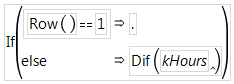Note: The Difference function also supports the Group By option.

Lag

Returns the value in the previous row for the selected column.

Lag Multiple

Returns the values from multiple previous rows for the selected column.

Cumulative Sum

Calculates the cumulative sum for each value in the selected column using the formula: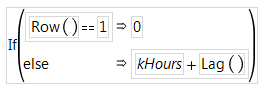Note: The Cumulative Sum function also supports the Group By option.

Moving Average

Calculates the exponentially weighted moving average, EWMA (using a smoothing parameter between 0 to 1.0) for each value in the selected column. The following example uses a smoothing parameter of 0.25: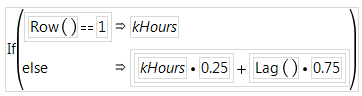Weighting

Determines how the values are weighted. Incremental weighting is a ramp or triangle. The exponential moving average is EWMA or EMA.

Items Before

Controls the size of the range (or window) by including the specified number of items before the current item in the average (in addition to the current item). -1 means all prior items.

Items After

Controls the size of the range (or window) by including the specified number of items after the current item in the average (in addition to the current item). -1 means all following items.

Report missing values for partial window

Controls how missing values are treated. By default, missing values are ignored.

Note: JMP evaluates the formula entered on-demand; complex formulas might require a lot of processing time.

Select a transform from the Vector menu to create transform columns from vector data.

To Columns

Expands the vector into individual number columns.

Sum

Returns the sum of the arguments or of the values within the vector.

Min

Returns the minimum value among the values within the vector.

Max

Returns the maximum value among the values within the vector.

Loc Min

Returns the first position of the minimum value.

Loc Max

Returns the first position of the maximum value.

### Transform Column Options

After creating a transform column, you can perform the following actions:

Rename

Renames the transform column.